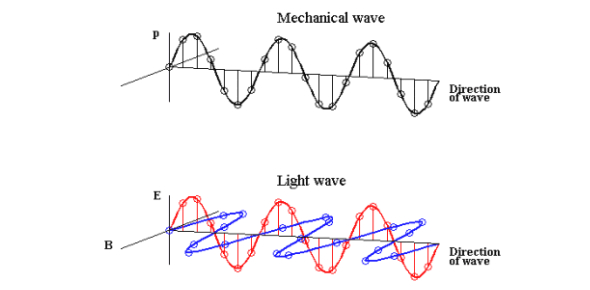# Mechanical Wave Test

10 Questions | Total Attempts: 1538SettingsA mechanical wave in the interesting physics (seriously) is a wave that transfers energy through a medium. Examples include sound waves and seismic waves. Not examples are humans or other living things. So, we can say the mediums are non-living yes?

• 1.
What is the difference between a mechanical and an electromagnetic wave?
• A.

Electro

• B.

Wave

• C.

Time

• D.

Medium

• 2.
Which is a type of mechanical wave?
• A.

Sun beam

• B.

Xrays

• C.

Transverse wave

• D.

Electro wave

• 3.
A parameter of mechanical wave include?
• A.

Ray

• B.

Water

• C.

Amplitude

• D.

Sound

• 4.
An example of mechanical wave is?
• A.

X ray

• B.

Water wave

• C.

Micro wave

• D.

Electromagnetic wave

• 5.
The highest point of a transverse wave is referred to as?
• A.

Frequency

• B.

Crest

• C.

Amplitude

• D.

Trough

• 6.
The distance between a subsequent crest and a trough is half of?
• A.

Wavelength

• B.

Frequency

• C.

Amplitude

• D.

Time

• 7.
The lowest point of a wave is known as?
• A.

Frequency

• B.

Crest

• C.

Trough

• D.

Amplitude

• 8.
How many parts has a transverse wave?
• A.

1

• B.

None

• C.

2

• D.

3

• 9.
The wavelength is the distance from crest to?
• A.

Trough

• B.

Crest

• C.

Node

• D.

Right angle

• 10.
The farthest distance apart in the longitudinal wave is the?
• A.

Crest

• B.

Wavelength

• C.

Rarefaction

• D.

Compression

Related Topics